## （数字图像处理MATLAB+Python）第十章图像分割-第三，四节：区域分割和基于聚类的图像分割

article2023/9/22 2:14:42/

• 一：区域分割
• （1）区域生长
• A：原理
• B：示例
• C：程序
• （2）区域合并
• A：原理
• B：示例
• C：程序
• （3）区域分裂
• A：原理
• B：示例
• C：程序
• （4）区域分裂合并
• A：原理
• B：示例
• 二：基于聚类的图像分割
• （1）原理
• （2）K均值聚类
• （3）程序

# 一：区域分割

• 区域生长
• 区域合并
• 区域分裂
• 区域合并分裂

## （1）区域生长

### A：原理

• 选择种子点： 首先从图像中选择一个或多个种子像素作为起始点。通常情况下，种子点可以通过人工选择或者根据一些特定规则自动确定（可以是单个像素，也可以是包括若干个像素的子区域，根据具体问题，利用先验知识来选择）
• 定义相似性准则： 确定像素之间的相似性度量标准，如灰度值、颜色、纹理等。通常，选择与种子像素相似度高于某个阈值的邻接像素
• 种子扩展： 从种子点开始，根据相似性准则逐渐添加邻接像素到当前区域中。可以使用递归、队列等方式来扩展种子区域
• 判断停止条件： 在区域生长的过程中，需要定义停止条件，即什么情况下停止合并。常见的停止条件包括达到一定区域大小、相似性度量低于阈值等
• 迭代： 重复执行种子扩展和判断停止条件的过程，直到所有的区域都被完全分割出来

### B：示例

• 种子点：(2,2)
• 相似性准则：灰度值差小于2
• 邻域选择：4领域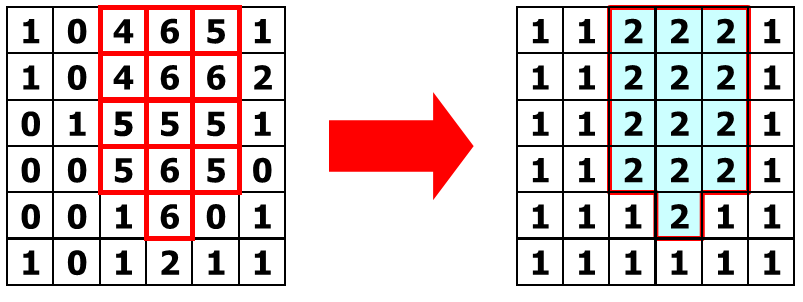### C：程序matlab实现

clear,clc,close all;
[height,width,channel]=size(Image);
if channel==3
Image=rgb2gray(Image);
end
figure,imshow(Image);
% Image=[1 0 4 6 5 1;1 0 4 6 6 2;0 1 5 5 5 1;0 0 5 6 5 0;0 0 1 6 0 1;1 0 1 2 1 1];
% [height,width,channel]=size(Image);
% figure,imshow(Image);
[seedx,seedy,button] = ginput(1);
seedx=round(seedx);
seedy=round(seedy);
region=zeros(height,width);
region(seedy,seedx)=1;
region_mean=Image(seedy,seedx);
region_num=1;
flag=zeros(height,width);
flag(seedy,seedx)=1;
neighbor=[-1 -1;-1 0;-1 1;0 -1;0 1;1 -1;1 0;1 1];
for k=1:8
y=seedy+neighbor(k,1);
x=seedx+neighbor(k,2);
waiting(k,:)=[y,x];
flag(y,x)=2;
end

pos=1;
len=length(waiting);
while pos<len
len=length(waiting);
current=waiting(pos,:);
pos=pos+1;
pixel=Image(current(1),current(2));
pdist=abs(pixel-region_mean);
if pdist<40/255
region(current(1),current(2))=1;
region_mean=region_mean*region_num+pixel;
region_num=region_num+1;
region_mean=region_mean/region_num;
for k=1:8
newpoint=current+neighbor(k,:);
if newpoint(1)>0 && newpoint(1)<=height && newpoint(2)>0 && newpoint(2)<width && flag(newpoint(1),newpoint(2))==0
waiting(end+1,:)=newpoint;
flag(newpoint(1),newpoint(2))=2;
end
end
end
end
figure,imshow(region),title('        ');
imwrite(region,'regiongrow.jpg');



python实现

import cv2
import numpy as np
import matplotlib.pyplot as plt

# 读取图像
height, width, channel = image.shape
if channel == 3:
image = cv2.cvtColor(image, cv2.COLOR_BGR2GRAY)

plt.imshow(image, cmap='gray')
plt.show()

# [seedx, seedy, button] = ginput(1) 在这里用鼠标点击代替，获取种子点的位置
seedx, seedy = np.round(plt.ginput(1))
seedx, seedy = int(seedx), int(seedy)
region = np.zeros((height, width))
region[seedy, seedx] = 1
region_mean = image[seedy, seedx]
region_num = 1
flag = np.zeros((height, width))
flag[seedy, seedx] = 1
neighbor = np.array([[-1, -1], [-1, 0], [-1, 1], [0, -1], [0, 1], [1, -1], [1, 0], [1, 1]])

waiting = []
for k in range(8):
y = seedy + neighbor[k, 0]
x = seedx + neighbor[k, 1]
waiting.append([y, x])
flag[y, x] = 2

pos = 0
while pos < len(waiting):
current = waiting[pos]
pos += 1
pixel = image[current, current]
pdist = abs(pixel - region_mean)
if pdist < 40 / 255:
region[current, current] = 1
region_mean = region_mean * region_num + pixel
region_num += 1
region_mean /= region_num
for k in range(8):
newpoint = current + neighbor[k]
if 0 < newpoint <= height and 0 < newpoint < width and flag[newpoint, newpoint] == 0:
waiting.append(newpoint)
flag[newpoint, newpoint] = 2

plt.imshow(region, cmap='gray')
plt.title('')
plt.show()

cv2.imwrite('regiongrow.jpg', region * 255)



## （2）区域合并

### A：原理

• 初始化： 将每个像素或小区域视为一个独立的区域。
• 相似性度量： 定义一种度量标准来衡量两个区域的相似性，这可以包括像素值、颜色、纹理、形状等特征。较低的相似性值意味着区域更相似。
• 合并条件： 设定一个合并条件，根据相似性度量来判断是否应该将两个相邻的区域合并。这可以是一个阈值，表示只有相似性高于阈值的区域才能合并。
• 区域合并： 从小区域开始，遍历图像的每个像素或区域，检查与相邻区域的相似性。如果两个相邻区域的相似性高于合并条件，则将它们合并成一个更大的区域。
• 迭代： 可能需要多次迭代才能达到最终的合并状态，因为合并一个区域可能会影响其他区域的合并条件

### B：示例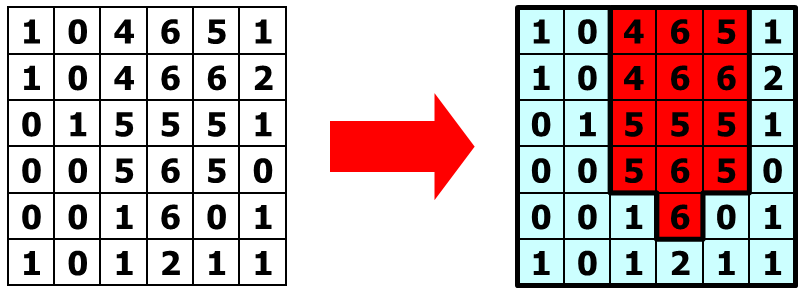### C：程序

• 初始化：每个像素为一个小区域；相似性准则采用：相邻区域灰度均值差≤2；左上角第一个点设为区域1，其余为0，表示未标记
• 第一次扫描图像：从左到右，从上到下，判断每一点与其左上、上、左邻点的灰度距离，三个距离中最小的若符合合并规则，将对应邻点的标记赋予当前点；若没有相似的点，则赋予当前点新的标记
• 再次扫描图像：若某一像素点上、左邻点标记不一致，但当前点和其中一个邻点标记一致，则判断两个区域是否是同一个，若是，则将两个区域标记修改为较小的一个，即区域合并

matlab实现

clear,clc,close all;

Image=[1 0 4 6 5 1;1 0 4 6 6 2;0 1 5 5 5 1;0 0 5 6 5 0;0 0 1 6 0 1;1 0 1 2 1 1];
[height,width,channel]=size(Image);
flag=zeros(height,width);
thresh=2;
neighbor=[-1 -1;-1 0;0 -1];
flag(1,1)=1;
number=1;
for j=1:height
for i=1:width
pdist=[300 300 300];
for k=1:3
y=j+neighbor(k,1);
x=i+neighbor(k,2);
if x>=1 && y>=1
pdist(k)=abs(Image(j,i)-Image(y,x));
end
end
[mindist,pos]=min(pdist(:));
if mindist<=thresh
y=j+neighbor(pos,1);
x=i+neighbor(pos,2);
if flag(y,x)
flag(j,i)=flag(y,x);
end
elseif mindist~=300
number=number+1;
flag(j,i)=number;
end
end
end

for j=2:height
for i=2:width
if flag(j-1,i)~=flag(j,i-1) && (flag(j,i)==flag(j-1,i) || flag(j,i)==flag(j,i-1))
pdist=abs(Image(j-1,i)-Image(j,i-1));
if pdist<=thresh
minv=min(flag(j-1,i),flag(j,i-1));
maxv=max(flag(j-1,i),flag(j,i-1));
flag(flag==maxv)=minv;
end
end
end
end


python实现

import numpy as np
import matplotlib.pyplot as plt

Image = np.array([[1, 0, 4, 6, 5, 1],
[1, 0, 4, 6, 6, 2],
[0, 1, 5, 5, 5, 1],
[0, 0, 5, 6, 5, 0],
[0, 0, 1, 6, 0, 1],
[1, 0, 1, 2, 1, 1]])

height, width = Image.shape
flag = np.zeros((height, width))
thresh = 2
neighbor = np.array([[-1, -1], [-1, 0], [0, -1]])
flag[0, 0] = 1
number = 1

for j in range(height):
for i in range(width):
pdist = [300, 300, 300]
for k in range(3):
y = j + neighbor[k, 0]
x = i + neighbor[k, 1]
if x >= 0 and y >= 0:
pdist[k] = abs(Image[j, i] - Image[y, x])
mindist, pos = min((val, idx) for (idx, val) in enumerate(pdist))
if mindist <= thresh:
y = j + neighbor[pos, 0]
x = i + neighbor[pos, 1]
if flag[y, x]:
flag[j, i] = flag[y, x]
elif mindist != 300:
number += 1
flag[j, i] = number

for j in range(1, height):
for i in range(1, width):
if flag[j - 1, i] != flag[j, i - 1] and (flag[j, i] == flag[j - 1, i] or flag[j, i] == flag[j, i - 1]):
pdist = abs(Image[j - 1, i] - Image[j, i - 1])
if pdist <= thresh:
minv = min(flag[j - 1, i], flag[j, i - 1])
maxv = max(flag[j - 1, i], flag[j, i - 1])
flag[flag == maxv] = minv

plt.imshow(flag, cmap='tab20')
plt.title('')
plt.show()



## （3）区域分裂

### A：原理

• 初始化： 将整幅图像或整个区域视为一个初始区域。
• 划分条件： 设定一个划分条件，根据某些特定的准则来判断是否应该将一个区域继续分割为子区域。这可能涉及到像素值、颜色、纹理、形状等特征。
• 区域分裂： 从初始区域开始，检查该区域是否满足划分条件。如果满足条件，则将该区域分割成更小的子区域。
• 递归： 对于每个子区域，如果满足继续划分的条件，继续对子区域进行递归分割，直到不再满足划分条件为止。
• 重复： 反复执行区域分裂和递归步骤，直到所有满足条件的区域都被分割为止

### B：示例

1：初始化及准则、方法的确定

• 区域内最大灰度值与最小灰度值之差 ≤ \leq 2
• 采用一分为四的分裂方法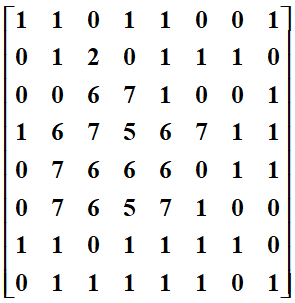2：采用一分为四的分裂方法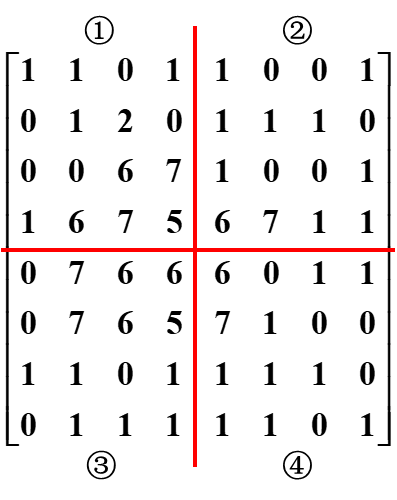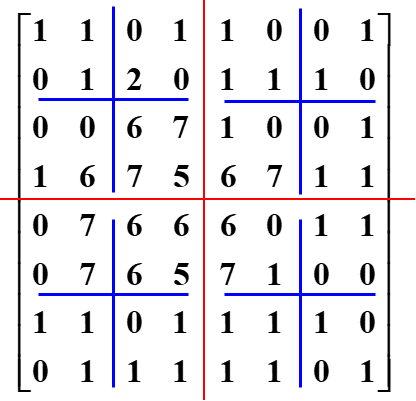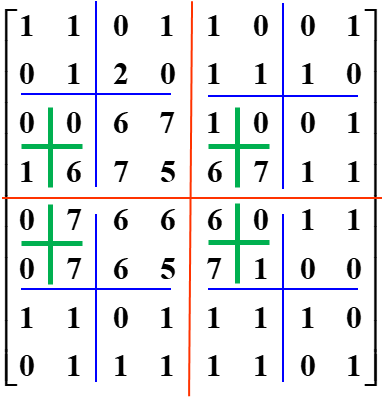### C：程序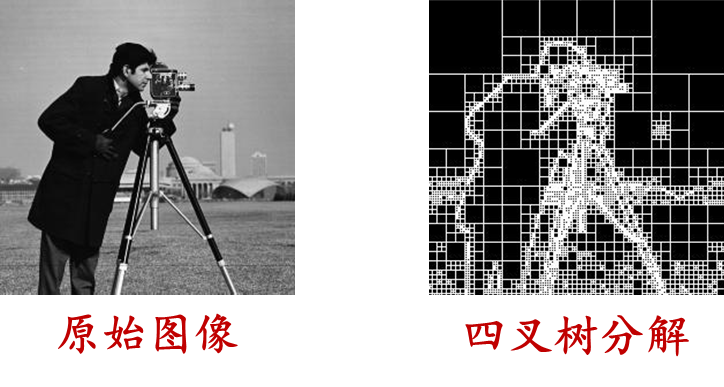matlab实现

Image=imread('cameraman.jpg');
S=qtdecomp(Image,0.27);
blocks=repmat(uint8(0),size(S));
for dim=[256 128 64 32 16 8 4 2 1]
numblocks=length(find(S==dim));
if(numblocks>0)
values=repmat(uint8(1),[dim dim numblocks]);
values(2:dim,2:dim,:)=0;
blocks=qtsetblk(blocks,S,dim,values);
end
end



python实现

import cv2
import numpy as np
import matplotlib.pyplot as plt

# 读取图像

S, _ = cv2.qr(Image, 0.27)

blocks = np.zeros_like(S, dtype=np.uint8)

for dim in [256, 128, 64, 32, 16, 8, 4, 2, 1]:
numblocks = np.sum(S == dim)
if numblocks > 0:
values = np.ones((dim, dim, numblocks), dtype=np.uint8)
values[1:dim, 1:dim, :] = 0
blocks = cv2.qt_set_block(blocks, S, dim, values)

plt.imshow(blocks, cmap='gray')
plt.title('')
plt.show()



## （4）区域分裂合并

### A：原理

• 初始分割： 将整幅图像划分成初始的小区域，每个区域可以是单个像素或者更大的局部区域
• 分裂过程： 首先，对每个区域进行分裂判断，判断条件可以是像素之间的相似性、颜色一致性等。如果一个区域不满足分裂条件，那么该区域将不再继续分裂，否则将该区域继续分裂成更小的子区域
• 合并过程： 在分裂得到的小区域中，判断相邻的区域是否满足合并条件，合并条件可以是相似性高于阈值等。如果相邻区域满足合并条件，将它们合并成更大的区域
• 迭代： 反复执行分裂和合并过程，直到没有进一步的分裂和合并可以执行为止，生成最终的分割结果

### B：示例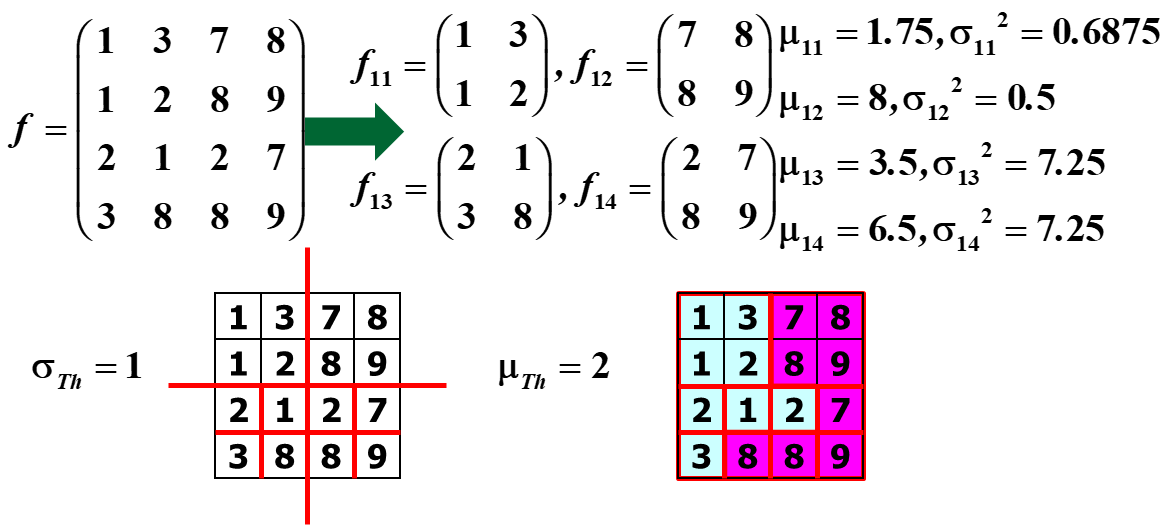# 二：基于聚类的图像分割

## （1）原理

：用向量来代表像素或像素周围邻域，向量的元素为与像素相关的特征，根据图像的具体情况，判断待分割区域的共性来设计

• 略（请查看聚类专栏）

## （3）程序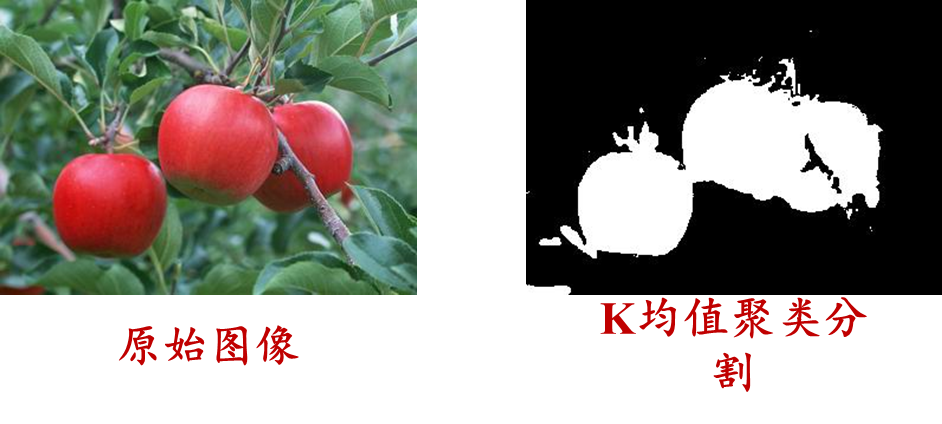matlab实现

clear,clc,close all;
imshow(Image);
hsv=rgb2hsv(Image);
h=hsv(:,:,1);
h(h>330/360)=0;
[N,M]=size(h);
training=h(:);
startdata = [0;60/360;120/360;180/360;240/360;300/360];
[IDX,C]= kmeans(training,6,'Start',startdata);
idbw = (IDX == 1);
template = reshape(idbw, size(h));
figure,imshow(template),title('分割后的图像');
imwrite(template,'clusterseg.jpg');



python实现

import cv2
import numpy as np
from sklearn.cluster import KMeans
import matplotlib.pyplot as plt

# 读取图像
Image = cv2.cvtColor(Image, cv2.COLOR_BGR2RGB)

# 显示原始图像
plt.imshow(Image)
plt.title('')
plt.show()

# 转换为HSV颜色空间并提取H通道
hsv = cv2.cvtColor(Image, cv2.COLOR_RGB2HSV)
h = hsv[:, :, 0]
h[h > 330] = 0  # 将大于330的值设为0

N, M = h.shape
training = h.reshape(-1, 1)
startdata = np.array([0, 60, 120, 180, 240, 300]) / 360

# 使用K均值聚类进行分割
kmeans = KMeans(n_clusters=6, init=startdata.reshape(-1, 1), n_init=1)
kmeans.fit(training)
labels = kmeans.labels_
centers = kmeans.cluster_centers_

idbw = (labels == 0)
template = idbw.reshape((N, M))

# 显示分割后的图像
plt.imshow(template, cmap='gray')
plt.title('')
plt.show()

cv2.imwrite('clusterseg.jpg', template * 255)



### tailscale使用教程（远程连接服务器）

tailscale&#xff1a;将多个设备放在同一局域网下&#xff0c;实现异地组网。 首先进入tailscale官网&#xff0c;根据系统需求进行下载 需要远程的设备和被远程的设备都需要下载。 然后两个设备均登录同一账号即可 注&#xff1a;这里重点讲一下linux操作系统上的操作&…

### 读SQL学习指南（第3版）笔记02_数据类型

1. 命令行工具 1.1. mysql -u root -p; 1.2. mysql&#xff1e; show databases; 1.3. mysql&#xff1e; use sakila; 1.4. mysql&#xff1e; SELECT now(); 1.4.1. now()是MySQL的内建函数 1.4.2. 返回当前日期和时间 1.5. mysql&#xff1e; SELECT now() FROM dual…

### 【数据结构入门指南】二叉树顺序结构: 堆及实现（全程配图，非常经典）

【数据结构入门指南】二叉树顺序结构: 堆及实现&#xff08;全程配图&#xff0c;非常经典&#xff09; 一、前言&#xff1a;二叉树的顺序结构二、堆的概念及结构三、堆的实现&#xff08;本篇博客以实现小堆为例&#xff09;3.1 准备工作3.2 初始化3.3 堆的插入3.3.1 向上调…

### 蓝凌OA custom.jsp 任意文件读取

​曾子曰&#xff1a;“慎终追远&#xff0c;民德归厚矣。” 漏洞复现 访问漏洞url&#xff1a; 出现漏洞的文件为 custom.jsp&#xff0c;构造payload&#xff1a; /sys/ui/extend/varkind/custom.jsp var{"body":{"file":"file:///etc/passwd&q…

### 代码随想录打卡—day24—【回溯】— 基础，最新820 8.21 todo

1 理论基础 回溯法也可以叫做回溯搜索法&#xff0c;它是一种搜索的方式。回溯算法——回溯和递归是相辅相成的。回溯法的效率&#xff0c;回溯法其实就是暴力查找&#xff0c;并不是什么高效的算法。回溯法解决的问题都可以抽象为树形结构&#xff08;N叉树&#xff09; 1.1…